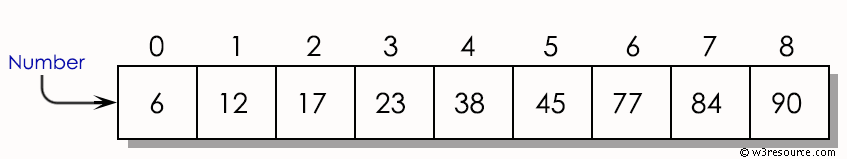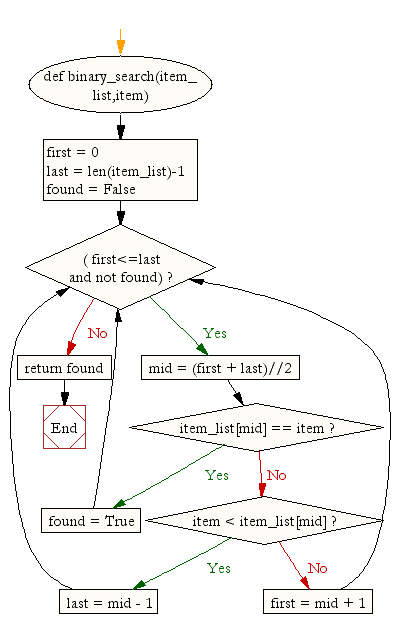﻿ Python Data Structures and Algorithms: Binary search - w3resource# Python Data Structures and Algorithms: Binary search

## Python Search and Sorting : Exercise-1 with Solution

Write a Python program for binary search.
Binary Search : In computer science, a binary search or half-interval search algorithm finds the position of a target value within a sorted array. The binary search algorithm can be classified as a dichotomies divide-and-conquer search algorithm and executes in logarithmic time.

Step by step example :Sample Solution:

Python Code:

``````def binary_search(item_list,item):
first = 0
last = len(item_list)-1
found = False
mid = (first + last)//2
if item_list[mid] == item :
found = True
else:
if item < item_list[mid]:
last = mid - 1
else:
first = mid + 1
return found

print(binary_search([1,2,3,5,8], 6))
print(binary_search([1,2,3,5,8], 5))
```
```

Sample Output:

```False
True
```

Flowchart:## Visualize Python code execution:

The following tool visualize what the computer is doing step-by-step as it executes the said program:

Python Code Editor :

What is the difficulty level of this exercise?

Test your Python skills with w3resource's quiz

﻿

## Python: Tips of the Day

Creating a sequence of numbers (zero to ten with skips):

```>>> range(0,10,2)
[0, 2, 4, 6, 8]
```